### Question 6. Solve the following simultaneous equation. 5x + 2y = –3; x + 5y = 4

Question 6.

Solve the following simultaneous equation.

5x + 2y = –3; x + 5y = 4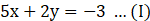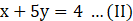Multiply Eq. I by 5 and Eq. II by 2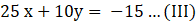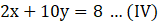Change sign of Eq.(IV)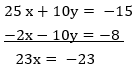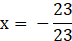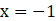Substituting x=–1in Eq.II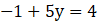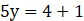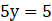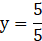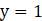∴ solution is (x, y) = (–1, 1)

MATHS I SSC NEW SYLLABUS

Chapter No. 1. Linear Equations in Two Variables

Practice Set 1.1

Practice Set 1.2

Practice Set 1.3

Practice Set 1.4

Practice Set 1.5

Problem Set No. 1

Practice Set 2.1

Practice Set 2.2

Practice Set 2.3

Practice Set 2.4

Practice Set 2.5

Practice Set 2.6

Problem Set No. 2

Chapter No. 3: Arithmetic Progression

Practice Set 3.1

Practice Set 3.2

Practice Set 3.3

Practice Set 3.4

Problem Set No 3

Chapter 4: Financial Planning.

Practice set 4.1

Practice set 4.2

Practice set 4.3

Practice set 4.4

Problem set 4 A

Problem set 4 B

CHAPTER NO. 5. PROBABILITY

PRACTICE SET 5.1

PRACTICE SET 5.4

PROBLEM SET NO. 5

Chapter No. 6: STATISTICS

PRACTICE SET 6.1

PRACTICE SET 6.2

PRACTICE SET 6.3

PRACTICE SET 6.4

PRACTICE SET 6.5

PRACTICE SET 6.6

PROBLEM SET NO. 6

BOARD PAPERS WITH COMPLETE SOLUTION

Mathematics Part - i Paper Pattern and Guidelines

SSC Maths I March 2019 Board Paper Solution 10th Standard 11th, March, 2019

GENERAL MATHS ALGEBRA BOARD PAPERS

MATHS I PRACTICE PAPER FOR SCHOOL PRELIMINARY EXAM. [AS PER NEW SYLLABUS]

Paper No. 1

Paper No. 2

Paper No. 3

ALGEBRA SSC OLD SYLLABUS

ARITHMETIC PROGRESSION

LINEAR EQUATIONS

PROBABILITY

STATISTICS ONE

STATISTICS TWO

EXTRA HOTS SUMS

FORMULA

ALGEBRAIC FORMULAE